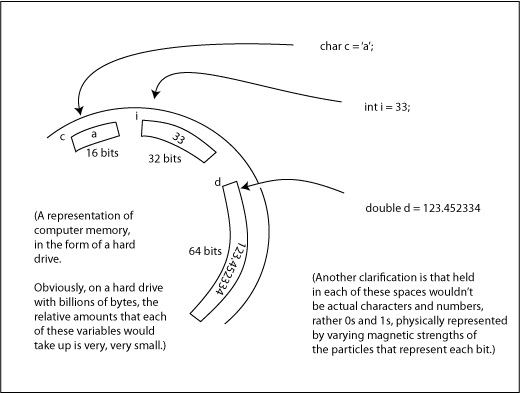Logout

More on Java Data Types

The reason for having various data types in a programming language like Java is very much in the interest of not wasting computer memory (i.e. the space where data is saved.) Different data types have different storage (i.e. memory) requirments. Consider a boolean versus a double. A double has to save very many digits, along with the position of the decimal point. Whereas all that the boolean has to store is true or false. And in fact, a double is called a double because it takes exactly double the amount of memory as the other real number type, the float.

We'll discuss what 'bits' and 'bytes' are in a bit more detail later on, but basically, a bit is the smallest division of computer memory on a device like a hard drive, or RAM. Here is a table that lists the amounts of memory (in terms of bits) that each of the common Java data types takes up:

 Type: # bits Data Example char 16 character 'a' String Depends on the number of chars in the string of chars string of characters "hello" short 16 integer 238 int 32 integer 1,999,234 long 64 integer 7,000,000,000 float 32 real number 3.14 double 64 real number 123.34234521 boolean 8 true/false true

Note that for the number types (short, int, long, float, and double), the Java types are "signed", meaning that they store both +ve and -ve values. That means that to calculate the range, you take 2 to the power of the number of bits, and half will be +ve and half will be -ve. So for example, for a signed 16 bit short (2 ^ 16 = 65,536), the range is from -32768 up to +32767.

So anyway, when we declare a variable as a certain data type, we are actually instructing the computer to go find the appropriate number of free bits, and reserve it for that variable. And from that point on while the program is running, that place will be referred to as our variable name.Some Other Points on Declaring Variables (of Certain Types)

- First of all, since part of the whole purpose of declaring variables of certain types is to reserve certain types of memory, you wouldn't (indeed, you can't) declare a variable over again, such as this:

int x = 44;
int x = 45; //<---Problem!!

You can't do this, since in the first line you already reserved the 32 bits for the int you are calling x. You don't need to do it again. If fact what Java thinks you are trying to do is reserve more space for another int called x, but you can't have two variables called the same name, as that would be confusing to the computer to know which one you were referring to. So the proper way, if you wanted to change the value of a variable is as follows:

int y = 88;
y = 89;

- chars are declared using single quotes, Strings with double quotes, and the other types with no quotes, as follows:

char c = '!';
String s = "Computer Science";
int i = 33;
float f = 1.2f;
double d = 1.22222222245;
boolean b = false;

- The names you use for your variables should be wisely chosen to represent the value. So if you were declaring a String that was the first name of a student, you would not call it s, as I have done in the basic examples, rather you'd write something like:

String firstName = "Bob";

Here are some other examples of good variable names:

int numberOfChairs = 17;

There are some rules that you must abide by in chosing variable names, like they can't start with a number, but the biggest one to recognize is that there can be no spaces in the name.

- Which brings us to the another point to note with naming variables; if there are more than one word that make up the variable name, capitalize the first letter of the second and so on words. But good convention is to not capitalize the very first letter of the variable name; that is typically reserved for the naming of classes only. So, for example:

boolean canGoOnTrip = true;
String homeworkName = "More On Java Data Types";

- But, finally, do be careful not to make your variable names too long, or so short you don't know what they mean. Here are some bad examples:

int theNumberIAmGoingToUseForChairNumber = 17; //<---Problematic!!
int chNum = 17; //<---Problematic!!
int chairNumber = 17; //<---Just right.